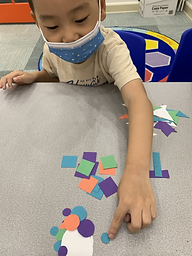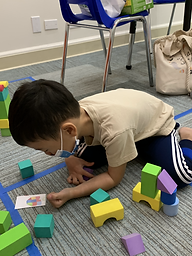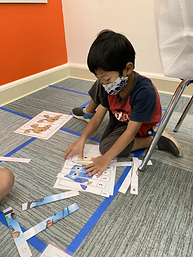Ms. Kayla

Target 1​

Lesson Type:

Continuation

Geometry

:

Shape

Identify and compare basic geometric shapes, regardless of orientation or size.

1:

Identify simple geometric shapes in a variety of colors, sizes, and orientations.

2:

Independently identify squares, rectangles, circles, and triangles.

3:

Identify basic geometric shapes that best represent real-world objects.

4:

Classify basic geometric shapes based on attributes.

Pre-K

Vocabulary:

Shape, Sort, Square, Triangle, Rectangle, Circle, Hexagon, Number, Trace

Activities:

1. Students started with sorting shapes into groups (circles, triangles, rectangles, squares).

2. Students identified and counted the number of shapes in a given picture.

3. Student then created a "shape monster".Home Exploration

Guiding Questions:Absent Students:

Target 2

:

1:

Use a 2D diagram as a guide or map to build something 3D.

2:

Use directional language to help describe how a 3D and 2D representations are the same (in the picture the red block is in front of the blue block).

Pre-K

Vocabulary:

Shape, Rectangle, Circle, Triangle, Square, Top, Bottom, Right, Left

Activities:

1. Students used images of structures to represent with 3D foam blocks.Home Exploration

Guiding Questions:Target 3

:

1:

Understand that the number sequence does not change, even when some numbers are missing from a given sequence.

2:

Identify when a given sequence is a correct count sequence.

Pre-K

Vocabulary:

Numbers, Before, After

Activities:

1. Students use picture puzzles with numbers to order numbers.Home Exploration